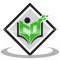## Is \$0.3 \$ the multiplicative inverse of \$3 \\frac{1}{3} \$ ? Why or why not?Updated on 10-Oct-2022 13:47:38

## Write the additive inverse of each of the following.(i) \$\\frac{2}{8} \$(ii) \$\\frac{-5}{9} \$(iii) \$\\frac{-6}{-5} \$(iv) \$\\frac{2}{-9} \$(v) \$\\frac{19}{-6} \$.Updated on 10-Oct-2022 13:47:38
To do:We have to write the additive inverse of the given rational numbers.Solution:Additive Inverse:The number in the set of real numbers that when added to a given number will give zero. (i) Let the additive inverse of the given rational number be $x$.Therefore, $x+\frac{2}{8}=0$$x=0-(\frac{2}{8})$$=0-\frac{2}{8}$   $=-\frac{2}{8}$The additive inverse of the given rational number is $-\frac{2}{8}$.    (ii) Let the additive inverse of the given rational number be $x$.Therefore, $x+\frac{-5}{9}=0$$x=0-(\frac{-5}{9})$$=0+\frac{5}{9}$   $=\frac{5}{9}$The additive inverse of the given rational number is $\frac{5}{9}$.     (iii) Let the additive inverse of the given rational number be $x$.Therefore, $x+\frac{-6}{-5}=0$$x=0-(\frac{-6}{-5})$$=0-\frac{6}{5}$   $=-\frac{6}{5}$The additive inverse of the given rational number is $-\frac{6}{5}$.      (iv) Let the additive ... Read More

## Using appropriate properties find.(i) \$-\\frac{2}{3} \\times \\frac{3}{5}+\\frac{5}{2}-\\frac{3}{5} \\times \\frac{1}{6} \$(ii) \$\\frac{2}{5} \\times\\left(-\\frac{3}{7}\\right)-\\frac{1}{6} \\times \\frac{3}{2}+\\frac{1}{14} \\times \\frac{2}{5} \$.Updated on 10-Oct-2022 13:47:38

## Find the mode of $14,25,14,28,18,17,18,14,23,22,14,18$.Updated on 10-Oct-2022 13:47:38
To do:We have to find the mode of $14,25,14,28,18,17,18,14,23,22,14,18$.Solution:To find the mode of the given data, we have to arrange the data in ascending order.The given data in ascending order is $14,14,14,14,17,18,18,18,22,23,25,28$In the given data,Frequency of $14$ is $4$Frequency of $17$ is $1$Frequency of $18$ is $3$Frequency of $22$ is $1$Frequency of $23$ is $1$Frequency of $25$ is $1$Frequency of $28$ is $1$We know that,Mode is the value or values in the data set that occur most frequently.Therefore,Mode of the given data is $14$.